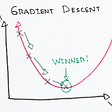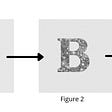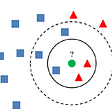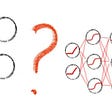# Feature Selection: Filter Methods

## 4 Filter-based methods to choose relevant features

Feature Selection is a very popular question during interviews; regardless of the ML domain. This post is part of a blog series on Feature Selection. Have a look at Wrapper (part2) and Embedded (part3) Methods.

# What, When & Why

Are you familiar with the Iris flower data set? Isn’t it amazing how wonderful results you can have with even the simplest algorithms that exist out there?

Well… I am sorry to disappoint you but this is not realistic. Most often, the number of features (p) is a lot more compared to the number of samples (N) (p>>N) — this is also known as the curse of dimensionality. But yet again, why is this a problem?

High dimensional data can lead to the following:

• long training times
• overfitting

Even if p>>N is not the case, there is a long list of machine learning algorithms that make the assumption of independent variables. Applying feature selection methods will remove correlated features. Additionally, reducing the dimensionality of the feature space to a subset of relevant features will decrease the computational cost of training and may improve the generalisation performance of the model.

Feature Selection is the process that removes irrelevant and redundant features from the data set. The model, in turn, will be of reduced complexity, thus, easier to interpret.

“Sometimes, less is better!”

— Rohan Rao

# Filter Methods

A subset of features is selected based on their relationship to the target variable. The selection is not dependent of any machine learning algorithm. On the contrary, filter methods measure the “relevance” of the features with the output via statistical tests. You can use the following table for reference:

## Pearson’s Correlation

A statistic that measures the linear correlation between two variables, which are both continuous. It varies from -1 to +1, where +1 corresponds to positive linear correlation, 0 to no linear correlation, and −1 to negative linear correlation.

Dataset: Boston Houses house-prices dataset. It includes 13 continuous features and the median value of owner-occupied homes in \$1000s (target variable).

`import pandas as pdimport matplotlib.pyplot as pltimport seaborn as snsfrom sklearn.datasets import load_bostonX, y = load_boston(return_X_y=True)feature_names = load_boston().feature_namesdata = pd.DataFrame(X, columns=feature_names)data['MEDV'] = y# compute pearson's rtarget_correlation = data.corr()[['MEDV']]# we only care about the target variableplt.figure(figsize=(7,5))sns.heatmap(target_correlation, annot=True, cmap=plt.cm.Reds)plt.show()`
`# extract the most correlated features with the output variabletarget_correlation[abs(target_correlation)>0.5].dropna()`

Correlation coefficients whose magnitude are between 0.5 and 0.7 indicate variables which can be considered moderately correlated, thus we set the threshold value to 0.5.

From the 13 features, there are only 3 that strongly correlate with the target (relevant features); RM, PTRATIO and LSTAT. However, we have only checked the correlation of each individual feature with the output variable. Since many algorithms, like Linear Regression, assume that the input features are uncorrelated, we must calculate the pearson’s r between those top 3 features.

`sns.heatmap(data.corr().loc[['RM', 'PTRATIO', 'LSTAT'], ['RM', 'PTRATIO', 'LSTAT']], annot=True, cmap=plt.cm.Reds)plt.show()`

RM and LSTAT are correlated with each other, thus we choose one of them (dropping ie RM is equivalent to removing redundant features). Since the correlation between LSTAT with target variable MEDV is higher than with RM, we select LSTAT.

## LDA

Linear Discriminant Analysis is a supervised linear algorithm that projects the data into a smaller subspace k (k < N-1) while maximising the separation between the classes. More specifically, the model finds linear combinations of the features that achieve maximum separability between the classes and minimum variance within each class.

Dataset: Breast Cancer Wisconsin (Diagnostic) Data Set that includes 569 records each described by 30 features. The task is classifying a tumor as malignant or benign.

`import pandas as pdfrom sklearn.linear_model import LogisticRegressionfrom sklearn.preprocessing import LabelEncoderfrom sklearn.discriminant_analysis import LinearDiscriminantAnalysisfrom sklearn.model_selection import StratifiedKFold, cross_val_scorefrom sklearn.pipeline import Pipelinedf = pd.read_csv('breast_cancer.csv').iloc[:,1:-1]X = df.drop(['diagnosis'], axis=1)le = LabelEncoder()y = le.fit_transform(df.diagnosis)labels = le.classes_steps = [('lda', LinearDiscriminantAnalysis()), ('m', LogisticRegression(C=10))]model = Pipeline(steps=steps)# evaluate modelcv = StratifiedKFold(n_splits=5)n_scores_lda = cross_val_score(model, X, y, scoring='f1_macro', cv=cv, n_jobs=-1)model = LogisticRegression(C=10)n_scores = cross_val_score(model, X, y, scoring='f1_macro', cv=cv, n_jobs=-1)# report performanceprint('f1-score (macro)\n')print('With LDA: %.2f' % np.mean(n_scores_lda))print('Without LDA: %.2f' % np.mean(n_scores))`

The performance improved by 4% with the use of LDA as a preprocessing step.

## ANOVA

Analysis of Variance is a statistical method that tests whether different input categories have significantly different values for the output variable. The `f_classif`method from `sklearn` allows for the analysis of multiple groups of data to determine the variability between samples and within samples, in order to gain information about the relationship between the dependent and independent variables (read more). For example, we might want to test two procedures to see which one performs better than the other in terms of revenue.

`from sklearn.feature_selection import f_classif, SelectKBestfs = SelectKBest(score_func=f_classif, k=5)X_new = fs.fit(X, y)`

Note: Previously, we just set k=5. What if it is not 5 and it is 4? We can fine tune the number of selected features by performing Grid-Search with k-fold Cross Validation

`from sklearn.model_selection import StratifiedKFold, GridSearchfrom sklearn.pipeline import Pipelinefrom sklearn.linear_model import LinearRegressioncv = StratifiedKFold(n_splits=5)pipeline = Pipeline(steps=[('anova',fs), ('lr', LinearRegression(solver='liblinear'))])params = {['anova__k']: [i+1 for i in range(X.shape)]}search = GridSearchCV(pipeline, params, scoring='accuracy', n_jobs=-1, cv=cv)results = search.fit(X, y)print('Best k: %s' % results.best_params_)`

## χ²

Chi-squared tests whether the occurrences of a specific feature and a specific class are independent using their frequency distribution. The null hypothesis is that the two variables are independent. However, large values of χ² indicate that the null hypothesis should be rejected. When selecting features, we wish to extract those that are highly dependent on the output.

Dataset: Dream Housing Finance company deals in all home loans and wishes to automate the loan eligibility process. The dataset contains 11 categorical and numerical features that describe a client’s profile. The target variable is binary — whether or not the client is eligible for loan.

`from sklearn.feature_selection import chi2, SelectKBestloan = pd.read_csv('loan_data_set.csv')loan = loan.drop('Loan_ID', axis=1) # irrelevant feature#Transform the numerical feature into categorical featureloan['Loan_Amount_Term'] = loan['Loan_Amount_Term'].astype('object')loan['Credit_History'] = loan['Credit_History'].astype('object')#Dropping all the null valueloan.dropna(inplace = True)#Retrieve all the categorical columns except the targetcategorical_columns = loan.select_dtypes(exclude='number').drop('Loan_Status', axis=1).columnsX = loan[categorical_columns].apply(LabelEncoder().fit_transform)y = LabelEncoder().fit_transform(loan['Loan_Status'])fs = SelectKBest(score_func=chi2, k=5)X_kbest = fs.fit_transform(X, y)`

So far, we have been discussing about methods that assume a linear relationship between two elements X, Y. Those methods fail to capture any relationship beyond that. To address this issue, we could have a look at the Mutual Information (MI) between the features and the target variable. MI ranges from 0 (no mutual information) and 1 (perfect correlation). Sklearn offers implementation for both regression and classification tasks.

`from sklearn.feature_selection import mutual_info_regression, mutual_info_classif, SelectKBestfs = SelectKBest(score_func=mutual_info_classif, k=5) # top 5 featuresX_subset = fs.fit_transform(X, y)`

You can read more about other ways to capture non-linear relationship between two variables here.

## Can I use Principal Component Analysis?

Of course you can. But please do not confuse Feature Extraction with Feature Selection. PCA is an unsupervised linear transformation technique. It is another way to reduce dimensionality — be careful though, in this method we do not choose features, we instead transform the feature space by projecting the data into a lower-dimensional space while preserving maximum variance. The technique results in uncorrelated variables (principal components) that are a linear combination of the old ones. Unfortunately, you don’t really know what the new features represent, so although you gain in dimensionality reduction you definitely lose on the interpretability.

Note: Do not make one of the most common mistake that young ML practitioners do: apply PCA on non-continuous features. I know that the code does not break when you run PCA on discrete variables but that does not mean that you should (short explanation).

## Feature Selection #NOT

Although we have seen plenty of ways to do feature selection (and there are more; check blog2, blog3), there is always the answer I wouldn’t do it”. I know that it might sound bizarre, especially when it comes from the author of this article, but I need to give all the possible answers and this is one of them.

Feature Selection takes time and you might consider not investing neither the time, nor the effort. You must always keep in mind of two things: 1. you will lose information since you are dropping features and 2. even if you try all the techniques, it can be that no major improvement is seen on the model’s performance.

--

--

--

## More from Analytics Vidhya

Analytics Vidhya is a community of Analytics and Data Science professionals. We are building the next-gen data science ecosystem https://www.analyticsvidhya.com

## Virus Xray Image Classification with Tensorflow Keras Python and Apache Spark Scala## The Key Points of Implementing Convolutional Neural Networks From Research Papers## Top 5 Natural Language Processing Python Libraries for Data Scientist.## Deploying ML Models on Azure## Mathematical Intuition behind Gradient Descent## Object detection and Deep Learning: Identify Google Streetview image content using YOLO in R## K-Nearest Neighbors from Scratch## Elli Tzini

ML Engineer with a passion for NLP

## One more approach to optimize neural networks## Creating scalable NLP pipelines using PySpark and Nlphose